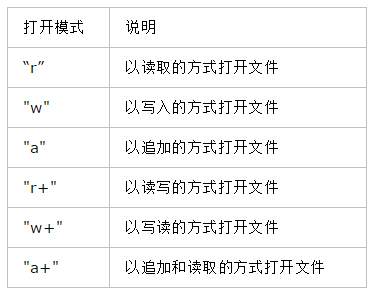python游戏开发：pygame中的IO、数据

1.输出

python一次可以打印多个变量，只要用一个逗号将每个变量隔开就可以了。比如：

A = 123
B = "ABC"
C = 456
D = "DEF"
print(A,B,C,D)

import sys
print(sys.platform)
print(sys.version)

2.获取用户的输入：

name = raw_input("Hi! what's your name?")
print(name)

3.异常处理

s = input("Enter a number: ")
try:
number = float(s)
except:
number = 0
print(number,"*",number,"=",answer)

1.操作文本文件：#调用open函数打开一个文件
file =open("data.txt","r")
#在完成操作后要将文件关闭
file.close()

file = open("data2.txt", "w")
file.write("abcdefg\n")
file.close()

text_lines = [
"abc\n",
"efg\n",
"hij\n" ]

file = open("data.txt", "w")
file.writelines(text_lines)
file.close()

file = open("data.txt", "r")

char = file.read(10)
print (char)

#这段代码会从文件中的当前指针位置读取10个字符
#像这样重复的调用，将继续从该文件读取更多的字符
#并且向前推进指针的位置

file = open("data.txt", "r")
print(all_data)
file.close()

print("Lines: ", len(all_data))
for line in all_data:
print(line.strip())

String.strip()方法会删去行末的换行字符。

2.操作二进制文件

 打开模式 说明 “rb” 以读取的方式打开二进制文件 "wb" 以写入的方式打开二进制文件 "ab" 以追加的方式打开二进制文件 "rb+" 以读取写入的方式打开二进制文件 "wb+" 以写入读取的方式打开二进制文件 "ab+" 以追加和读取的方式打开二进制文件

1.写入二进制文件：

import struct

file = open("bin.dat", "wb")
for n in range(100):
data = struct.pack('i', n)
file.write(data)
file.close()

#这段代码是将100个整数写入到文件中

2.读取二进制文件

file = open("bin.dat", "rb")
size = struct.calcsize("i")
file.close()
struct.calcsize("i")可以计算出一个int类型的大小，以便让struct.unpack知道应该读取多少个字节的数据。